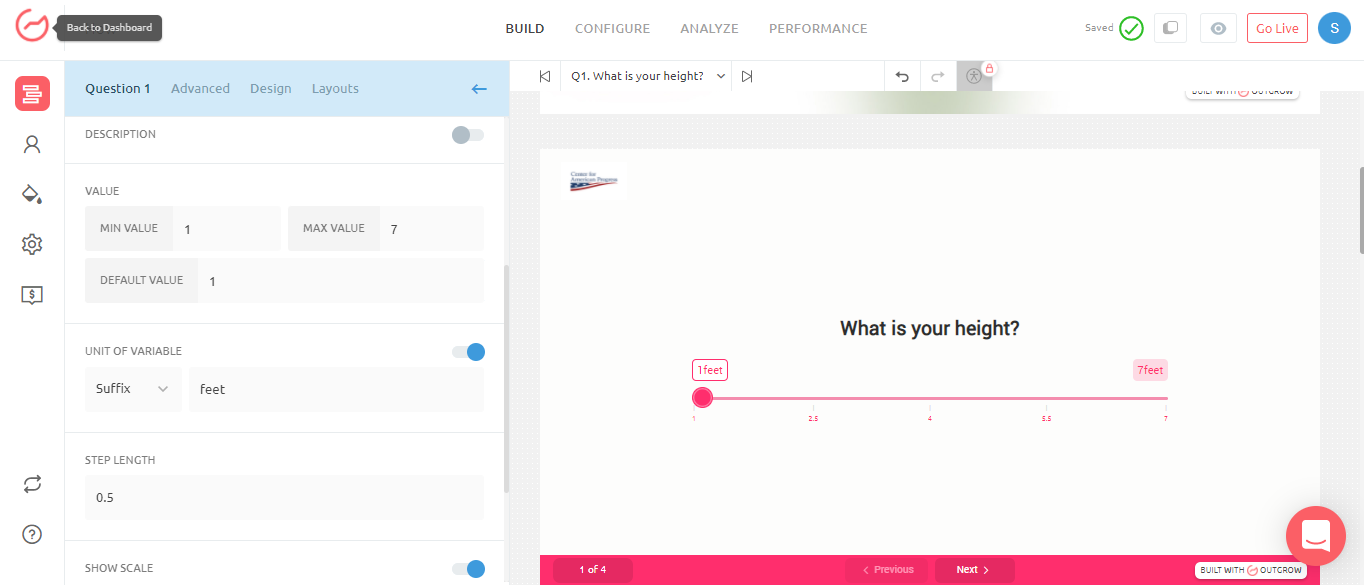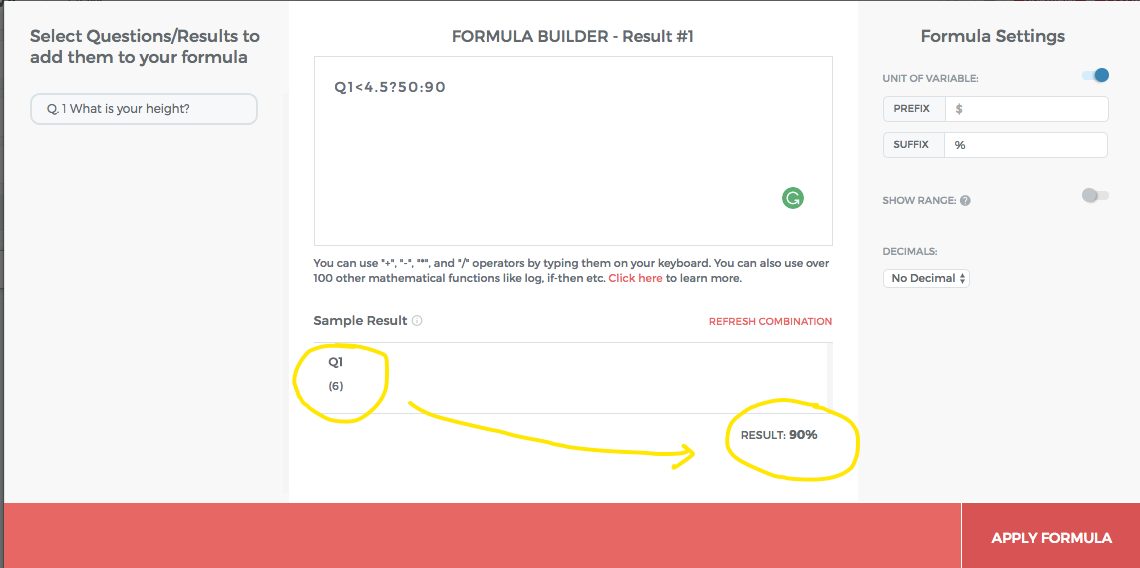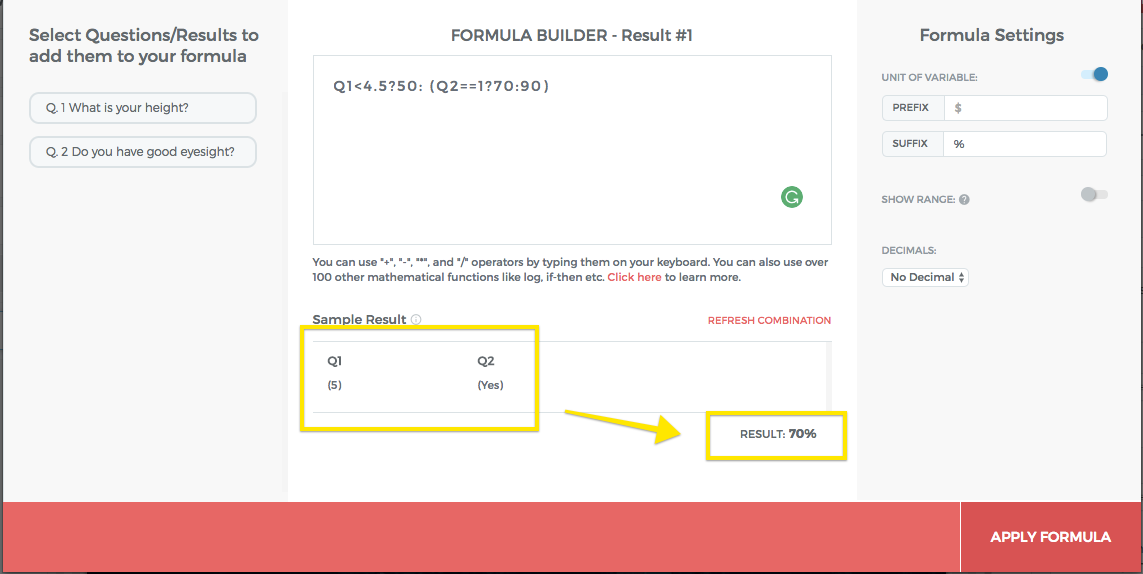# How to Create a Calculator Using Conditional Logic?

You can use basic operators like +, -, x and / by typing them out on the keyboard. You can easily build simple cost and savings calculators with these basic operators. If you would like to use advanced operators like ^ and log, you can use them too. Simply type them out on your keyboard.

However, if you would like to use more complicated formulas, you can read the sections below.

# Using If-Then-Else Statements

To use conditional expressions, you need to use a formula of type x ? y : z. Let's use it in an example to better understand:

Say we are trying to access the risk of you hitting your head on the ceiling and it depends on your height. So you will ask a question "What is your height?" and on the basis of the answer assign a risk value.The expression will take the form: If my height is < 4.5 feet then my risk of hitting my head on the roof is 50%, else it is 90%. To write this expression is the formula builder, just write:

Q1 < 4.5 ? 50 : 90
*Here, "?" becomes the expression for "then", and ":" becomes the expression for "else".# Using Else-If Statements

This was a simple example but in a real situation, it may be more complicated. Say there was another question that asked if you have good eye sight. [if eyesight is good, them we assign a value of 1, else 0]So if a person's height is greater than 4.5 feet but has good eyesight, then their risk is 70% (if they have bad eyesight, then risk is 90%).

In this case, we will need to use a nested if else statement to account for the new question. Here is what it will look like.

Q1 < 4.5 ? 50 : (Q2 == 1 ? 70 : 90)

This statement can be paraphrased as: if height is <4.5 feet, the risk is 50%, but if height > 4.5 feet and eyesight is good, then risk os 70%, else it is 90%### 📘So on and so forth

Just like this, you can create as many else if statements to account for more variables and complications.

### ❗️Feel free to reach out if you need more help.

In case the documentation below doesn't answer your questions about your formula, feel free to email [email protected]. Many of us are mathematics majors and will be delighted to help :)

# [Advanced] Other Operators

For other advanced operators, please see the documentation for operators here and functions here.

Feel free to email [email protected] if you need any help with the formula. We will be delighted to help :)